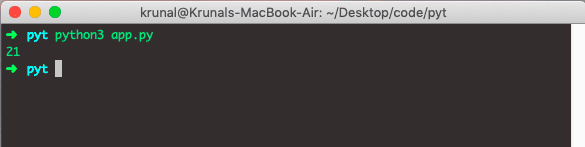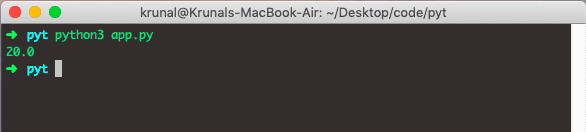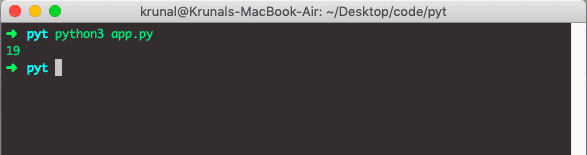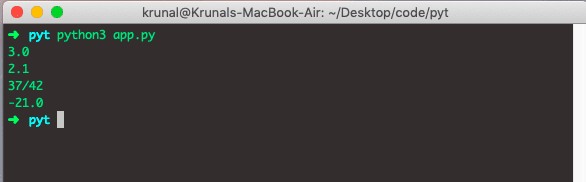# Python Median: How To Find Median of List

0
149## Python median()

The median() is a built-in math function in Python used to calculate the median value from an unsorted data list. To calculate the median in Python, you can use the statistics.median() function.

The most significant advantage of using the median() method is that the data list does not need to be sorted before being sent as a parameter to the median() function.

## How to find the median in Python

To calculate the median in Python, you can use the statistics.median() function. The following is a statistical formula to calculate the median of any dataset.

```Median = {(n + 1) / 2}th Value
```

The statistics median is the quick measure to find the data sequence’s central location, list, or iterator. Python statistics.median() function returns the median (middle value) of numeric data.

Note:

When the number of data points in the given sequence or list or iterator is odd, an exact middle data point (number) is returned.

## Python Median of list

To find the median of the list in Python, we can use statistics.median() method. The list can be of any size, and the numbers are not guaranteed to be in a particular order.

If the list contains an even number of items, the function should return an average of the middle two. Python 3.4 has `statistics.median `function.

The list can be of any size, and the numbers are not guaranteed to be in any particular order. If you are looking for a function that calculates the median() in Python 3, then the statistics.median() function is the solution.

The median() function returns the median (middle value) of numeric data.

When several data points are odd, return the middle data point. When the number of data points is even, a median is interpolated by taking the average of the two middle values.

Median is a value that separates a higher half of the data or probability distribution from the lower half. If the list contains an even number of elements, the function should return the middle two average.

When the number of items in the list or tuple or any iterator is odd, it returns the middle data point.

If the number of data points in the list or tuple is even, the median is interpolated by taking an average of the two middle values.

Okay, let’s define a list with the odd number of items.

```# app.py

import statistics

listA = [19, 46, 21, 18, 30]
print(statistics.median(listA))```

In the above-written code, you can see that 21 is the median number, and you can run the above file and check the output in the console.Now, let’s find a median where the list contains an even number of items.

```# app.py

listA = [6, 46, 21, 19, 18, 30]
print(statistics.median(listA))```

See the output.We can find the median of any dataset that can be list or tuple or iterable with a set of numeric values. If the items are empty or null, then StatisticsError is raised.

## Finding the median of a tuple in Python

To calculate the median of a tuple in Python, we can use statistics.median() method. Let’s define a tuple and then find its median.

```# app.py

import statistics

tupleA = (21, 19, 19, 21, 18)
print(statistics.median(tupleA))```

See the output.In the above code, first, we have imported the statistics module, and then we have used the median() function to find the median of the list.

Let’s see another example.

Write the following code inside the app.py file.

```# app.py

import statistics
from fractions import Fraction as fr

data1 = (1, 2, 2, 3, 3, 3, 4, 4, 4, 4)
print(statistics.median(data1))

data2 = (2.1, 1.9, 2.1, 1.8, 2.9)
print(statistics.median(data2))

data3 = (fr(19, 21), fr(18, 21), fr(19, 21), fr(21, 46))
print(statistics.median(data3))

data4 = (-21, -22, -21, -29, -18, -19)
print(statistics.median(data4))```

See the output.## StatisticsError in median() function

If we pass the empty list in the median() function, it will return a StatisticsError. Okay, we get the StatisticsError if the list is empty. See the following code.

```# app.py

import statistics

data = []

print(statistics.median(data))

```

See the following output.

```➜  pyt python3 app.py
Traceback (most recent call last):
File "app.py", line 5, in
print(statistics.median(data))
File "/Library/Frameworks/Python.framework/Versions/3.6/lib/python3.6/statistics.py", line 380, in median
raise StatisticsError("no median for empty data")
statistics.StatisticsError: no median for empty data
➜  pyt
```

From the StatisticsError, you can say that no median for empty data.

## Using the numpy module

We can also compute the median() method using the numpy module.  In that case, we don’t need the statistics module.

```# app.py

import numpy as np

dataset = [21, 19, 11, 21, 19, 46, 29]
op = np.median(dataset)

print(op)
```

See the following output.

```➜  pyt python3 app.py
21.0
➜  pyt
```

Any value in the dataset at an abnormal distance from all the other values can be termed the outlier.

Outliers generally tend to skew a mean radically.

Outliers can be present in a dataset with a very high value or with a deficient value.

So, the median is the value that lies at the center.

## Conclusion

Median is described as the middle number when all numbers are sorted from smallest to largest. In simple translation, sort all numbers in a list from the smallest one to the largest one. Whichever number is in the middle is the median.

In case there are even several items in a data set, a median is an average of the two values that lie in the center.

Finally, Python Median Function Example is over.